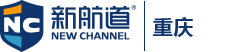4001859090

## 新航道名师解析：A-level数学等差数列模块真题

2019-12-24 10:09 来源：重庆编辑Find the sum of the arithmetic progression 1,4,7,10,13,16,….1000

Every third term of the above progression is removed, i.e. 7,16, ect. Find the sum of the remaining terms.

1000=1+(n-1)3 → n=334

S=½×334(1+1000)=167167

a1=7, d1=9，一共有334项，假设移出了约三分之一 111

l1=7+(111-1）×9=997

S1=½×111(7+997)=55722

S2=S-S1=167167-55722=111445

+86

+86

### 收不到验证码？

• 1.请检查是否输入正确的手机号码
• 2.检查手机是否停机
• 3.请使用其他账号登录
• 4.请联系官方客服

+86